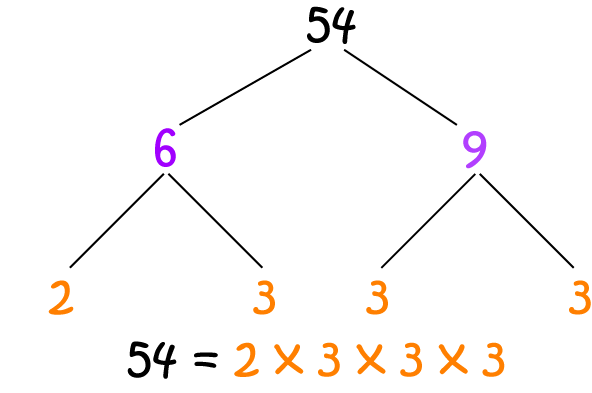# Definition of Prime FactorA prime factor is a factor of a number that is a prime number.

In other words, a prime factor of a number is a prime number that can be multiplied by an integer to give the original number.

You can find prime factors of every number. So, every number can be written as a product of its prime factors.

For example, the prime factors of $14$ are $2$ and $7$, because $14 = 2 \times 7$.

For example, the prime factors of $54$ are $2$ and $3$, because $54 = 2 \times 3 \times 3 \times 3$.

### Description

The aim of this dictionary is to provide definitions to common mathematical terms. Students learn a new math skill every week at school, sometimes just before they start a new skill, if they want to look at what a specific term means, this is where this dictionary will become handy and a go-to guide for a student.

### Audience

Year 1 to Year 12 students

### Learning Objectives

Learn common math terms starting with letter P

Author: Subject Coach
You must be logged in as Student to ask a Question.